#### Exercise 2.

Factor the polynomial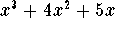completely (a) over the real numbers, (b) over the complex numbers.

We can first pull out an x term: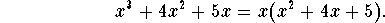The polynomial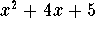has the roots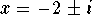by the quadratic formula. It is thus irreducible over the real numbers. Consequently, over the real numbers, the polynomial factors completely as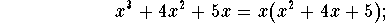over the complex numbers, the polynomial factors completely into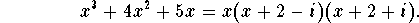[Back] [Exercises] [Next]
[Algebra] [Trigonometry] [Complex Variables]
[Calculus] [Differential Equations] [Matrix Algebra]S.O.S MATHematics home page

Do you need more help? Please post your question on our S.O.S. Mathematics CyberBoard.Helmut Knaust
Tue Jun 24 09:45:58 MDT 1997